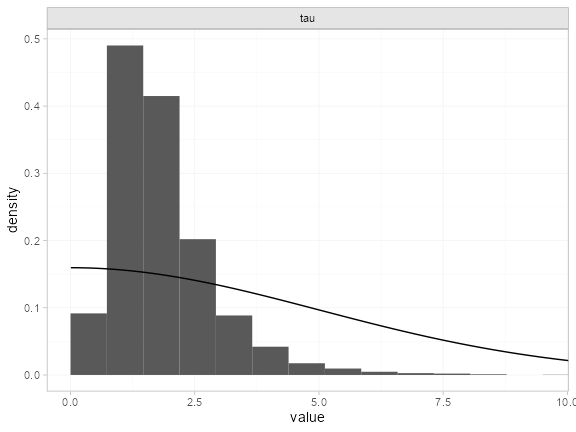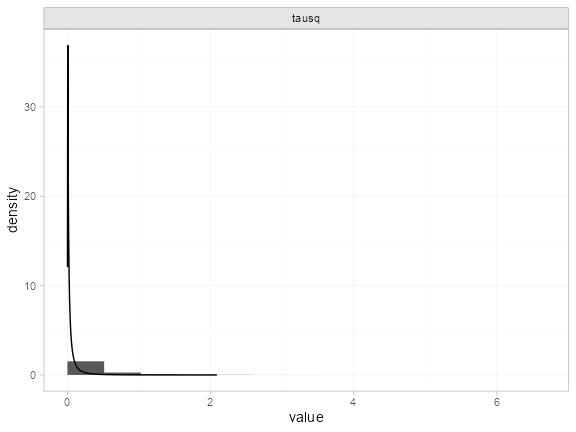# Example: White blood cell transfusion

library(multinma)
options(mc.cores = parallel::detectCores())

This vignette describes the analysis of 6 trials comparing transfusion of granulocytes (white blood cells) to control for preventing mortality in patients with neutropenia or neutrophil dysfunction . The data are available in this package as transfusion:

head(transfusion)
#>        studyc        trtc  r  n
#> 1    Bow 1984 Transfusion  5 13
#> 2    Bow 1984     Control  4 11
#> 3 Herzig 1977 Transfusion  1 13
#> 4 Herzig 1977     Control  3 14
#> 5  Higby 1975 Transfusion  2 17
#> 6  Higby 1975     Control 14 19

previously used this dataset to demonstrate the application of informative priors for heterogeneity, an analysis which we recreate here.

## Setting up the network

We begin by setting up the network - here just a pairwise meta-analysis. We have arm-level count data giving the number of deaths (r) out of the total (n) in each arm, so we use the function set_agd_arm(). We set “Control” as the reference treatment.

tr_net <- set_agd_arm(transfusion,
study = studyc,
trt = trtc,
r = r,
n = n,
trt_ref = "Control")
tr_net
#> A network with 6 AgD studies (arm-based).
#>
#> ------------------------------------------------------- AgD studies (arm-based) ----
#>  Study         Treatment arms
#>  Bow 1984      2: Control | Transfusion
#>  Herzig 1977   2: Control | Transfusion
#>  Higby 1975    2: Control | Transfusion
#>  Scali 1978    2: Control | Transfusion
#>  Vogler 1977   2: Control | Transfusion
#>  Winston 1982a 2: Control | Transfusion
#>
#>  Outcome type: count
#> ------------------------------------------------------------------------------------
#> Total number of treatments: 2
#> Total number of studies: 6
#> Reference treatment is: Control
#> Network is connected

## Meta-analysis models

We fit two random effects models, first with a non-informative prior for the heterogeneity, then using the informative prior described by .

### Random effects meta-analysis with non-informative heterogeneity prior

We fit a random effects model using the nma() function with trt_effects = "random". We use $$\mathrm{N}(0, 100^2)$$ prior distributions for the treatment effects $$d_k$$ and study-specific intercepts $$\mu_j$$, and a non-informative $$\textrm{half-N}(5^2)$$ prior for the heterogeneity standard deviation $$\tau$$. We can examine the range of parameter values implied by these prior distributions with the summary() method:

summary(normal(scale = 100))
#> A Normal prior distribution: location = 0, scale = 100.
#> 50% of the prior density lies between -67.45 and 67.45.
#> 95% of the prior density lies between -196 and 196.
summary(half_normal(scale = 5))
#> A half-Normal prior distribution: location = 0, scale = 5.
#> 50% of the prior density lies between 0 and 3.37.
#> 95% of the prior density lies between 0 and 9.8.

Fitting the RE model

tr_fit_RE_noninf <- nma(tr_net,
trt_effects = "random",
prior_intercept = normal(scale = 100),
prior_trt = normal(scale = 100),
prior_het = half_normal(scale = 5))

Basic parameter summaries are given by the print() method:

tr_fit_RE_noninf
#> A random effects NMA with a binomial likelihood (logit link).
#> Inference for Stan model: binomial_1par.
#> 4 chains, each with iter=2000; warmup=1000; thin=1;
#> post-warmup draws per chain=1000, total post-warmup draws=4000.
#>
#>                   mean se_mean   sd    2.5%     25%     50%     75%   97.5% n_eff Rhat
#> d[Transfusion]   -1.13    0.03 0.96   -3.23   -1.64   -1.10   -0.55    0.73  1195    1
#> lp__           -134.26    0.09 2.96 -141.17 -136.06 -133.91 -132.11 -129.30  1103    1
#> tau               1.84    0.03 1.03    0.54    1.15    1.61    2.27    4.55  1348    1
#>
#> Samples were drawn using NUTS(diag_e) at Thu Feb 24 09:05:43 2022.
#> For each parameter, n_eff is a crude measure of effective sample size,
#> and Rhat is the potential scale reduction factor on split chains (at
#> convergence, Rhat=1).

By default, summaries of the study-specific intercepts $$\mu_j$$ and study-specific relative effects $$\delta_{jk}$$ are hidden, but could be examined by changing the pars argument:

# Not run
print(tr_fit_RE_noninf, pars = c("d", "mu", "delta"))

The prior and posterior distributions can be compared visually using the plot_prior_posterior() function:

plot_prior_posterior(tr_fit_RE_noninf, prior = "het")The posterior distribution for the heterogeneity variance $$\tau^2$$ is summarised by

noninf_tau <- as.array(tr_fit_RE_noninf, pars = "tau")
noninf_tausq <- noninf_tau^2
names(noninf_tausq) <- "tausq"
summary(noninf_tausq)
#>       mean  sd 2.5%  25%  50%  75% 97.5% Bulk_ESS Tail_ESS Rhat
#> tausq 4.47 6.2 0.29 1.33 2.59 5.16 20.66     1368     1765    1

### Random effects meta-analysis with informative heterogeneity prior

Keeping the rest of the model setup the same, we now use an informative $$\textrm{log-N}(-3.93, 1.51^2)$$ prior for the heterogeneity variance $$\tau^2$$. We can examine the range of parameter values implied by this prior distribution with the summary() method:

summary(log_normal(-3.93, 1.51))
#> A log-Normal prior distribution: location = -3.93, scale = 1.51.
#> 50% of the prior density lies between 0.01 and 0.05.
#> 95% of the prior density lies between 0 and 0.38.

Fitting the RE model, we specify the log_normal prior distribution in the prior_het argument, and set prior_het_type = "var" to indicate that this prior distribution is on the variance scale (instead of the standard deviation, the default).

tr_fit_RE_inf <- nma(tr_net,
trt_effects = "random",
prior_intercept = normal(scale = 100),
prior_trt = normal(scale = 100),
prior_het = log_normal(-3.93, 1.51),
prior_het_type = "var")

Basic parameter summaries are given by the print() method:

tr_fit_RE_inf
#> A random effects NMA with a binomial likelihood (logit link).
#> Inference for Stan model: binomial_1par.
#> 4 chains, each with iter=2000; warmup=1000; thin=1;
#> post-warmup draws per chain=1000, total post-warmup draws=4000.
#>
#>                   mean se_mean   sd    2.5%     25%     50%     75%   97.5% n_eff Rhat
#> d[Transfusion]   -0.77    0.01 0.44   -1.72   -1.02   -0.73   -0.47    0.01  2405    1
#> lp__           -140.27    0.07 2.75 -146.58 -142.00 -139.95 -138.26 -135.85  1377    1
#> tau               0.47    0.01 0.35    0.05    0.20    0.40    0.67    1.32  1605    1
#>
#> Samples were drawn using NUTS(diag_e) at Thu Feb 24 09:05:55 2022.
#> For each parameter, n_eff is a crude measure of effective sample size,
#> and Rhat is the potential scale reduction factor on split chains (at
#> convergence, Rhat=1).

By default, summaries of the study-specific intercepts $$\mu_j$$ and study-specific relative effects $$\delta_{jk}$$ are hidden, but could be examined by changing the pars argument:

# Not run
print(tr_fit_RE_inf, pars = c("d", "mu", "delta"))

The prior and posterior distributions can be compared visually using the plot_prior_posterior() function:

plot_prior_posterior(tr_fit_RE_inf, prior = "het")Note: The heterogeneity variance $$\tau^2$$ is plotted here since the prior was specified on $$\tau^2$$.

The posterior distribution for the heterogeneity variance $$\tau^2$$ is summarised by

inf_tau <- as.array(tr_fit_RE_inf, pars = "tau")
inf_tausq <- inf_tau^2
names(inf_tausq) <- "tausq"
summary(inf_tausq)
#>       mean  sd 2.5%  25%  50%  75% 97.5% Bulk_ESS Tail_ESS Rhat
#> tausq 0.34 0.5    0 0.04 0.16 0.45  1.73     1490     3055    1